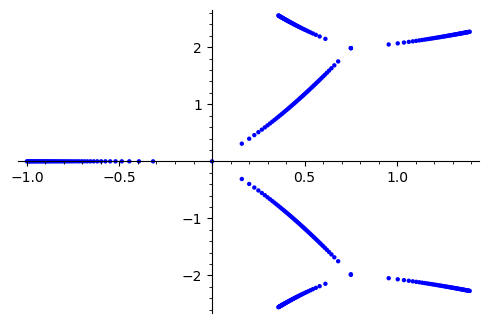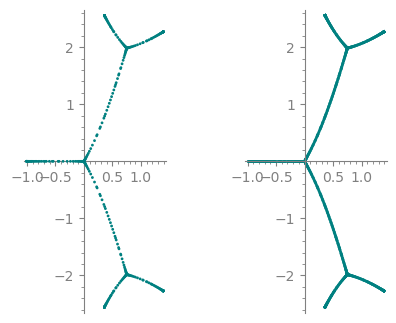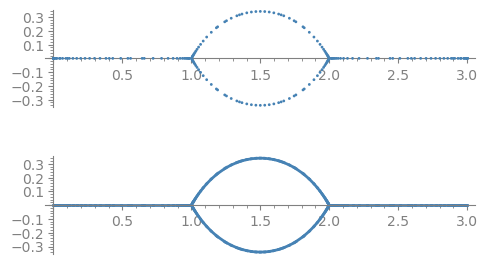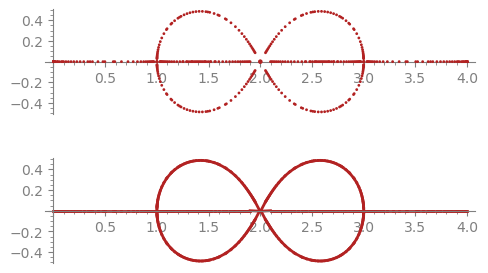2021-01-19 17:33:44 -0600 received badge ● Nice Answer (source) 2021-01-19 02:06:55 -0600 answered a question Print scaling factors in eta-products Like this sage: e = EtaGroup(8).basis() sage: e Eta product of level 8 : (eta_2)^-4 (eta_4)^12 (eta_8)^-8 sage: e._rdict {2: -4, 4: 12, 8: -8}  2021-01-16 04:57:59 -0600 commented question Removing all supersets of a given subset from a powerset? Adding elements one by one, you need to define a successor function : see https://doc.sagemath.org/html/en/refe... 2021-01-16 01:35:41 -0600 commented question Removing all supersets of a given subset from a powerset? Maybe using RecursivelyEnumeratedSet ? 2021-01-13 10:24:55 -0600 answered a question How to compute in the Tensor Algebra $T(V)$ ? Like this maybe sage: A = algebras.Free(QQ, 'abc') sage: elt = 4 * A.monomial(Word('abc')) + 6*A.monomial(Word('a')) sage: data = [(w.to_word(), cf) for w, cf in elt] sage: A.sum_of_terms((w[1:] + w[:1], cf) for w, cf in data) 6*a + 4*b*c*a  2021-01-11 11:43:37 -0600 commented question Trigonometric equation Use solve([tan(x) + tan(2*x) - tan(3*x) == 0], x, to_poly_solve='force', algorithm='sympy') 2021-01-11 06:19:29 -0600 edited question Problems with modules after installation Hello everyone, I have installed Sage on Ubuntu 16.04 from the pre-built tarball binary exactly as described in the installation guide but I am left with some newbie questions. After browsing the web for several hours, I still cannot manage to get the module graphs up and running in the terminal. First I couldn't even import graphs, but after running sage --pip install graphs in terminal I can do sage: import graphs  without ModuleNotFound error, but the command sage: G = graphs.EmptyGraph()  leads to AttributeError: module 'graphs' has no attribute 'EmptyGraph'  Moreover, I'd like to add a new package (sage-drg by Janos Vidali, which I cannot link) but it remains unclear on how to do this. Where do I unpack the zip? How do I let Sage know where to find this package? Is this even possible if I didn't built from source? 2021-01-11 03:32:27 -0600 commented question Problems with modules after installation Just in case, you may like to use sage: from sage.graphs.all import * 2021-01-11 03:31:05 -0600 commented question Problems with modules after installation There is no need to install any package, as graphs are included in sage itself. You have probably installed https://pypi.org/project/graphs/ which has nothing to do with sage. 2021-01-10 04:32:13 -0600 commented question Not able to generate a simple list of polynomial. I am getting list of list. You can use parent(t) to know in what t lives. In your case, each element of the list is a matrix. 2021-01-09 02:16:08 -0600 commented answer Translating quiver in QPA to Sage Have a look at sage: U.set_latex_options? 2021-01-08 14:22:49 -0600 commented answer Translating quiver in QPA to Sage Arrows names are there but not displayed by default. You need to ask for them to be displayed.sage: U.plot(edge_labels=True) 2021-01-08 14:18:51 -0600 received badge ● Nice Answer (source) 2021-01-08 13:11:23 -0600 answered a question Translating quiver in QPA to Sage you can just copy-paste if you want sage: data = ["v1","v2","v3","v4","v5","v6","v7","v8"], [["v1","v1","a"],["v1"," ....: v2","b"],["v2","v3","c"],["v3","v4","d"],["v4","v5","e"],["v5","v5","f"], ....: ["v3","v6","g"],["v6","v7","h"],["v7","v8","i"],["v8","v3","j"]] sage: DiGraph(data, format="vertices_and_edges",loops=True) Looped digraph on 8 vertices  EDIT: here is a more procedural way def from_QPA(Q): arrows = [(v.SourceOfPath(), v.TargetOfPath(), v) for v in Q.ArrowsOfQuiver() ] elements = Q.VerticesOfQuiver() return DiGraph([elements, arrows], loops=True, format='vertices_and_edges')  then sage: libgap.LoadPackage("QPA") true sage: Q = libgap.Quiver( ["v1","v2","v3"], [["v1","v2","a1"],["v2","v3","a2"],["v3","v1","a3"]] ) sage: from_QPA(Q) Looped digraph on 3 vertices  2021-01-01 05:10:40 -0600 commented question bug? multiply symbolic exponents but sage: (e^x)^y - e^(x*y) y ⎛ x⎞ x⋅y ⎝ℯ ⎠ - ℯ sage: simplify(_) 0  2021-01-01 05:08:34 -0600 edited question bug? multiply symbolic exponents Is this a bug ? sage: var('a b c x y z') (a, b, c, x, y, z) sage: assert( (e^x)^y == e^(x*y) ) --------------------------------------------------------------------------- AssertionError Traceback (most recent call last) /Users/marks/mysage/sage-9.0/local/lib/python3.7/site-packages/sage/all_cmdline.py in () ----> 1 assert( (e**x)**y == e**(x*y) ) AssertionError: sage: assert( (e^2)^3 == e^(2*3) ) sage:  2020-12-27 13:52:44 -0600 commented question Translating a list of polynomials using sage Side remark : sage: P.is_cyclotomic_product() is useful to recognize polynomials with all their roots on the unit circle. 2020-12-21 03:57:45 -0600 answered a question Implicit plot with complex function Are you working on dessins d'enfants? Here is a simple idea for an approximate plot. sage: x = polygen(QQ, 'x') sage: f = 1/729*(2*x**2-3*x+9)**3*(x+1) sage: N = 50 sage: point2d(root for k in range(N+1) for root in (f - k / N).complex_roots())The function pre01 below builds on this idea. It gives a good idea of the desired plot and runs fast. def pre01(f, n=50, style='dots', **opt): r""" Return the preimage of the unit interval under this rational function. INPUT: - f -- a rational function - n -- optional (default: 50) -- roughly how many points in the unit interval [0, 1] to use for preparing the plot Roughly a third or the points are inverse powers of two, roughly a third are one minus inverse powers of two, and roughly a third are linearly spaced along the interval. EXAMPLES:: sage: x = polygen(QQ, 'x') sage: f = 1/729 * (2 * x**2 - 3 * x + 9)**3 * (x + 1) sage: pre01(f, n=50, style='dots', color='firebrick').show(figsize=5) sage: pre01(f, n=50, style='line', color='firebrick').show(figsize=5) sage: z = polygen(QQ, 'z') sage: num = -(z^4-6*z^3+12*z^2-8*z)*(z-1)^3*(z-3) sage: den = (2*z-3)*(z-2)^3*z sage: g = num/den sage: pre01(g, n=50, style='dots', color='firebrick').show(figsize=5) sage: pre01(g, n=50, style='line', color='firebrick').show(figsize=5) sage: Qz. = QQ[] sage: cc = [81, -432, 972, -1200, 886, -400, 108, -16, 1] sage: num = -Qz(cc)*(z-2)^6*(z-4)*z sage: den = (6*z^4-48*z^3+140*z^2-176*z+81)*(z-1)^4*(z-3)^4 sage: h = num/den sage: pre01(h, n=50, style='dots', color='firebrick').show(figsize=5) sage: pre01(h, n=50, style='line', color='firebrick').show(figsize=5) """ nn = n // 3 + 2 num, den = f.numerator(), f.denominator() tt =  + [ZZ(2)**-k for k in (0 .. nn)] tt.extend([1 - t for t in tt[3:]]) tt.extend(QQ((k, nn)) for k in (1 .. nn - 1)) tt = sorted(set(tt)) cols = {'color': 'teal', 'axes': True, 'axes_color': 'grey', 'axes_label_color': 'grey', 'tick_label_color': 'grey'} dop = {'aspect_ratio': 1, 'size': 4, 'zorder': 10} lop = {'aspect_ratio': 1, 'edge_thickness': 2} for k, v in opt.items(): if 'color' in k or 'axis' in k or 'axes' in k or 'tick' in k: cols[k] = v elif k == 'size': dop[k] = v elif k == 'edge_thickness': lop[k] = v else: dop[k] = lop[k] = v if style == 'dots': zz = (root for t in set(tt) for root in (num - t*den).complex_roots()) G = point2d(zz, color=cols['color'], **dop) elif style == 'line': tzz = {t: (num - t*den).complex_roots() for t in tt} D = Graph() for k, t in enumerate(tt[1:-1], start=1): s, u = tt[k-1], tt[k+1] szz, uzz = tzz[s], tzz[u] for z in tzz[t]: zs = min((abs(z - w), w) for w in szz) D.add_edge(z, zs, k-1) zu = min((abs(z - w), w) for w in uzz) D.add_edge(z, zu, k) D.set_pos({z: (z.real(), z.imag()) for z in D}) ecol = {cols['color']: D.edges()} G = D.plot(vertex_labels=False, vertex_size=0, edge_colors=ecol, **lop) else: raise ValueError(f"style should be 'dots' or 'line', got :'{style}'") G.axes(cols['axes']) G.axes_color(cols['axes_color']) G.axes_label_color(cols['axes_label_color']) G.tick_label_color(cols['tick_label_color']) return G  Three examples of using this function follow. Quick starting example of this answer: x = polygen(QQ, 'x') f = 1/729 * (2 * x**2 - 3 * x + 9)**3 * (x + 1) a_dots = pre01(f, n=50, style='dots', color='teal') a_line = pre01(f, n=50, style='line', color='teal') a_dots_lines = graphics_array([a_dots, a_line], ncols=2) a_dots_lines.show(figsize=5)First example in the question: z = polygen(QQ, 'z') num = -(z^4-6*z^3+12*z^2-8*z)*(z-1)^3*(z-3) den = (2*z-3)*(z-2)^3*z b_dots = pre01(num/den, n=50, style='dots', color='steelblue') b_line = pre01(num/den, n=50, style='line', color='steelblue') b_dots_lines = graphics_array([b_dots, b_line], ncols=1) b_dots_lines.show(figsize=5)Second example in the question: z = polygen(QQ, 'z') num = -(z^8-16*z^7+108*z^6-400*z^5+886*z^4-1200*z^3+972*z^2-432*z+81)*(z-2)^6*(z-4)*z den = (6*z^4-48*z^3+140*z^2-176*z+81)*(z-1)^4*(z-3)^4 c_dots = pre01(num/den, n=50, style='dots', color='firebrick') c_line = pre01(num/den, n=50, style='line', color='firebrick') c_dots_lines = graphics_array([c_dots, c_line], ncols=1) c_dots_lines.show(figsize=5)Each of those three examples runs in under a second per plot style. 2020-12-20 10:48:02 -0600 commented question Does Sage support tensor of two symmetric functions? Here is a start sage: S=SymmetricFunctions(QQ).s() sage: S().tensor(S()) s # s  2020-12-17 15:26:33 -0600 received badge ● Nice Answer (source) 2020-12-17 05:00:58 -0600 commented question minimize_constrained works for f(x,y)=x+y but not for f(x,y)=x I have created https://trac.sagemath.org/ticket/31065 2020-12-17 04:13:31 -0600 commented question minimize_constrained works for f(x,y)=x+y but not for f(x,y)=x This is a bug, indeed. Comes from using "f.variables()" in minimize_constrained. 2020-12-17 01:43:55 -0600 commented question edge label in graph doesn't print The documentation says that you need to ask for the edge labels : G.show(edge_labels=True) 2020-12-16 05:04:22 -0600 commented question Positioning the nodes of a graph Read the documentation ? 2020-12-06 05:08:41 -0600 edited question 9.2 download for Ubuntu 20.04 documentation I've downloaded the Sage 9.2 Ubuntu 20.04 tarball and got it up and running. I'm having problems accessing the help documentation when I have a jupyter notebook open. When I click on any help topic, there is an error message: "404 Not Found. The page you are requesting does not exist" How can I fix this? Thanks for any help. 2020-12-05 01:53:51 -0600 edited question How to show lines and images on a chess board (matrix_plot) Hi, I naively created a chess board with a matrix_plot. m = matrix_plot([[1,0,1,0,1,0,1,0], [0,1,0,1,0,1,0,1], [1,0,1,0,1,0,1,0], [0,1,0,1,0,1,0,1], [1,0,1,0,1,0,1,0], [0,1,0,1,0,1,0,1], [1,0,1,0,1,0,1,0], [0,1,0,1,0,1,0,1], [1,0,1,0,1,0,1,0]] )  Shown alone, it looks almost like a chess board, but it has axes and white padding on the periphery  (m).show(aspect_ratio=1)  I then naively tried to add a line to that plot - Q = line([(-0.5,0),(1,1)]) (m+Q).show()  Somehow I have lost the whole matrix plot . This is version 8.7 in a jupyter notebook Q = line([(-0.5,0),(1,1)]) (m+Q).show()  i only get 2 rows - the rest is truncated. Question - how to graph lines on top of this matrix plot without truncating the apprearance? Merci! Pat 2020-12-02 12:08:59 -0600 commented question Downloading thousands of plots from a server sage notebook? Suggestion : find in which directory your notebook files lives, and un-archive them (they are just compressed archives). You may see the pictures there. 2020-12-02 01:33:50 -0600 commented answer Posets with infinite outer automorphism group You can accept my answer, maybe ? Also consider sponsoring sage : https://github.com/sponsors/sagemath 2020-11-30 10:07:52 -0600 commented question Composition of piecewise function This documentation link is obsolete and not official. Use only doc.sagemath.org 2020-11-30 09:26:44 -0600 commented question Some 2-d plot questions For 1, use P+Q where P and Q are two plots. Or use a sequence of functions as argument of plot. 2020-11-30 04:35:16 -0600 answered a question Posets with infinite outer automorphism group Some code for the r-number, not tested. def r_number(P): H = P.hasse_diagram() M = Matroid(H.to_undirected()) b = FreeModule(GF(2), tuple(H.edges(labels=False))).basis() vecs = [sum(b[e] for e in ci).to_vector() for ci in M.circuits()] return matrix(vecs).rank()  Remains only to do the same thing for the subspace that defines s. EDIT: here is a tentative, not tested either def s_number(P): H = P.hasse_diagram() b = FreeModule(GF(2), tuple(H.edges(labels=False))).basis() vecs = [] for x, y in P.relations(): chains = H.all_paths(x, y, report_edges=True) if len(chains) <= 1: continue v0 = sum(b[e] for e in chains).to_vector() vecs += [v0 + sum(b[e] for e in ci).to_vector() for ci in chains[1:]] return matrix(vecs).rank()  2020-11-30 04:20:51 -0600 commented question 'method' object is not subscriptable G.edges is a method, use list(G.edges()) for the list of edges. Store this in a variable before the loop. 2020-11-30 02:39:35 -0600 commented question Posets with infinite outer automorphism group Can this be computed using some kind of homology group ? 2020-11-29 12:13:19 -0600 received badge ● Nice Answer (source) 2020-11-29 06:31:37 -0600 answered a question Filtering certain posets Maybe something like this sage: def banana(n): ....: for P in posets(n): ....: if not P.is_connected(): ....: continue ....: H = P.hasse_diagram() ....: if H.to_undirected().is_tree(): ....: continue ....: dg = H.transitive_closure() ....: if any(dg.degree(x) == n - 1 for x in dg): ....: continue ....: yield P  2020-11-29 02:08:34 -0600 commented question name 'input_box' is not defined 2020-11-28 14:42:57 -0600 commented question How do you load a file to the SageMath 9.1 Console? 2020-11-28 13:11:55 -0600 commented question How do you load a file to the SageMath 9.1 Console? try load(r"C:\Users\vika\Desktop\example.sage") ? 2020-11-28 12:02:56 -0600 edited question What is next_probable_prime ? In my laptop for 200 digit numbers sage: time p=next_prime(10^200) CPU times: user 1.04 s, sys: 591 µs, total: 1.04 s Wall time: 1.04 s sage: time p=next_probable_prime(10^200) CPU times: user 20.7 ms, sys: 0 ns, total: 20.7 ms Wall time: 21.8 ms  What return exacty next_probable_prime? When is desired to use it? 2020-11-27 01:54:06 -0600 answered a question How to find sum of all elements of a matrix in sagemath Like this sage: M = matrix(2, 2, [1, 2, 3, 4]) sage: sum(M.coefficients()) 10  2020-11-26 02:17:36 -0600 commented question power series method pade(m,n) off by 1? I propose a fix here : https://trac.sagemath.org/ticket/30965 2020-11-26 01:45:28 -0600 commented question power series method pade(m,n) off by 1? Please look at the code and tell us where it is wrong. Open-source, we are.